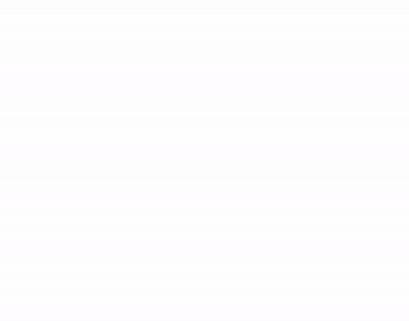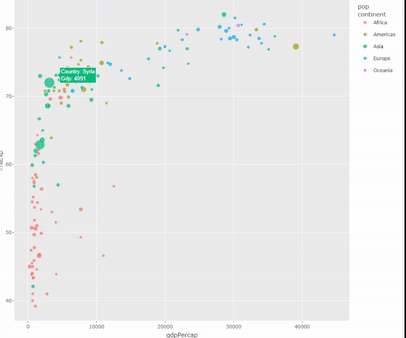Open in App
Not now

# Interactive Bubble Chart in R

• Last Updated : 14 Feb, 2023

A bubble chart is used to find and show relationships between numeric variables. with this technique, the dataset is visualized in two to four dimensions. It is used to find various insights into business and industry sectors. This chart is implemented in R Programming Language using Highcharter Library. Highcharter is an Open-Source library, which provides user-friendly interactive charts for the program as well as for web applications.

`Example: Creating Interactive Bubble Chart Fruits Left After Selling among the countries.`

### Creating Interactive Bubble Chart using highcharter

Load the dataset and import the Highcharter library. Create a chart object using hchart function. Pass type, respected data vectors, and Size of bubble, to the hchart(type, axes, max size), that needed to be plotted.

## R

 `# Creating dataframe for chart.``countries = ``c``(``'India'``, ``'Usa'``, ``'Canada'``,``              ``'Malaysia'``, ``'Japan'``)``apples = ``c``(1000, 3000, 2500, 6500, 5000)``mangoes = ``c``(100, 200, 300, 450, 600)``left_fruits = ``c``(10, 20, 30, 40, 50)``df = ``data.frame``(countries, apples, mangoes,``              ``left_fruits)` `# Importing Library``library``(highcharter)` `# Creating Chart Object.``chart <- df %>% ``hchart``(``'scatter'``,``                       ``hcaes``(x = apples,``                             ``y = mangoes,``                             ``size = left_fruits),``                       ``maxSize = ``"10%"``)``chart`

Output:Interactive Bubble Chart for Sample Dataset

### Creating Interactive Bubble Chart using ggplot2 and plotly

ggplot2 Package in R programming Language also termed as the grammar of graphics is free open source and easy to use. ggplot2 will make only graphs. to make them interactive we use Plotly library.

Load the gapminder dataset and filter the data needed to be displayed on the graph. Prepare a tooltip that needed to be displayed on the graph and pass the x-axis, y-axis, size, attribute, and tooltip to the ggplot(aes(x, y, size, color, text)). For displaying the relationship select the geom_point() function. Make the plot interactive using ggplotly(data, tooltip).

## R

 `# Libraries``library``(ggplot2)``library``(dplyr)``library``(plotly)` `# The dataset is provided in the gapminder library``library``(gapminder)``data <- gapminder %>% ``filter``(year==``"2002"``)``    ``%>% dplyr::``select``(-year)``data` `# Rounding the values for tooltip``data <- data %>%``  ``mutate``(gdpPercap=``round``(gdpPercap,0)) %>%``  ``mutate``(pop=``round``(pop/1000000,2)) %>%``  ``mutate``(lifeExp=``round``(lifeExp,1)) %>%` `# Text tooltip to display``  ``mutate``(text = ``paste``(``"Country: "``,``                      ``country,``"\nGdp: "``,``                      ``gdpPercap, sep=``""``)) %>%``  ` `# Classic ggplot``  ``ggplot``(``aes``(x=gdpPercap,``             ``y=lifeExp,``             ``size = pop,``             ``color = continent,``             ``text=text)) +``  ``geom_point``(alpha=0.7)`  `# Turn ggplot interactive with plotly``chart <- ``ggplotly``(data, tooltip=``"text"``)``chart`

Output:Interactive Bubble Chart for gapminder dataset

My Personal Notes arrow_drop_up